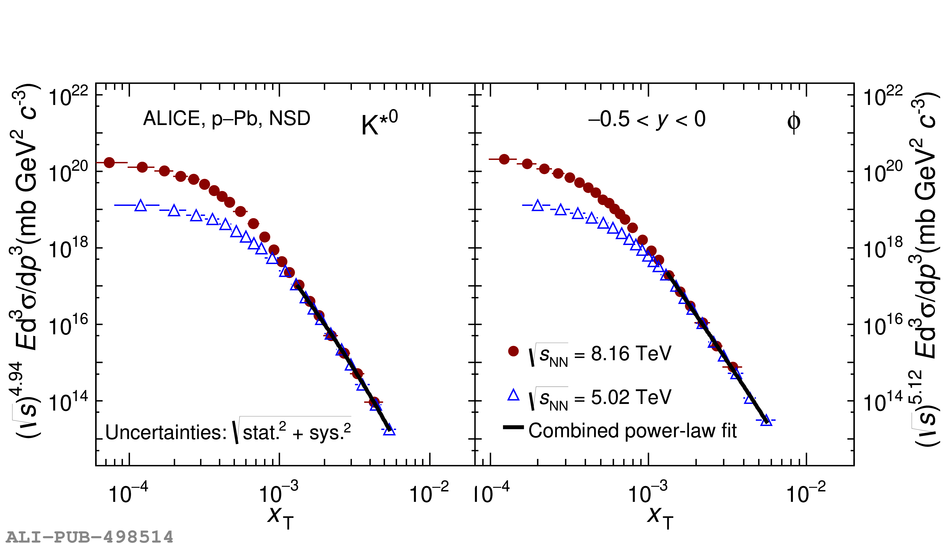Figure 7

 Scaled invariant yield of \kstar and \phim as a function of $x_{\rm{T}}$ = 2$p_{\mathrm{T}}/\snn$ in p--Pb collisions at different energies \snn = 5.02 and 8.16 TeV.